Class and Object in Python

View more Tutorials: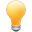Follow us on our fanpages to receive notifications every time there are new articles.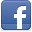Facebook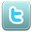Twitter

1- Object Oriented in Python

Python is a procedural-oriented programming language, and it also an object oriented programming language.
Procedural Oriented
"Procedural-oriented" is expressed in using functions in Python. You can define functions, and the functions can be used in other modules in the Python program.
Object Oriented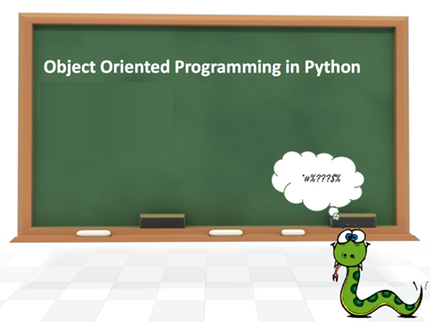"Object-oriented" in Python is expressed in using class, you can define a class, class is a prototype to create objects.

2- Create class in Python

The syntax for creating a class:
** class syntax **

class ClassName:
'Brief description of the class (Optional)'
# Code ...
• To define a class you use the class keyword, followed by the name of the class and the colon (:). The first line in the class body is a string that briefly describes this class. (Optional), you can access this string via Class__.Doc__
• In the class body, you can declare  attributes, methods, and constructors.
Attribute:
The attribute is a member of the class. For example, the rectangle has two attributes including width and height.
Method:
• The method of class is similar to a normal function but it is a function of class, in order to use it, you need to call through object.
• The first parameter of the method is always self (a keyword that refers to the class itself).
Constructor:
• Constructor is a special method of class, which is always named __init__.
• The first parameter of the constructor is always self (a keyword refers to the class itself).
• Constructor is used to create an object.
• Constructor assigns values of the parameter to the properties of the object that will be created.
• You can only define a constructor in class.
• If the class is not defined by the constructor, Python assumes that it has a default constructor, __init __ (self), with the null body.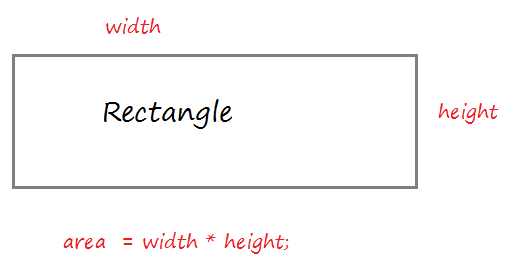rectangle.py

# Rectangle.
class Rectangle :
'This is Rectangle class'

# Method to create object (Constructor)
def __init__(self, width, height):

self.width= width
self.height = height

def getWidth(self):
return self.width

def getHeight(self):
return self.height

# Method to calculate Area.
def getArea(self):

return self.width * self.height
Create an object from the Rectangle class: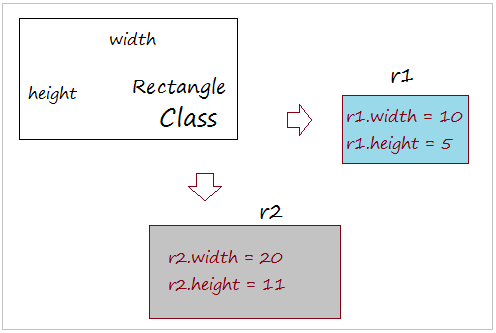testRectangle.py

from rectangle import Rectangle

# Create 2 objects: r1 & r2
r1 = Rectangle(10,5)

r2 = Rectangle(20,11)

print ("r1.width = ", r1.width)
print ("r1.height = ", r1.height)
print ("r1.getWidth() = ", r1.getWidth())
print ("r1.getArea() = ", r1.getArea())

print ("-----------------")

print ("r2.width = ", r2.width)
print ("r2.height = ", r2.height)
print ("r2.getWidth() = ", r2.getWidth())
print ("r2.getArea() = ", r2.getArea())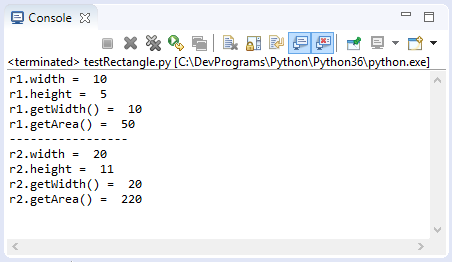What happens when you create an object from a class?
When you create an object of the Rectangle class, the constructor method of that class will be called to create an object, and the properties of the object will be assigned the value from the parameter. It is similar to the illustration below: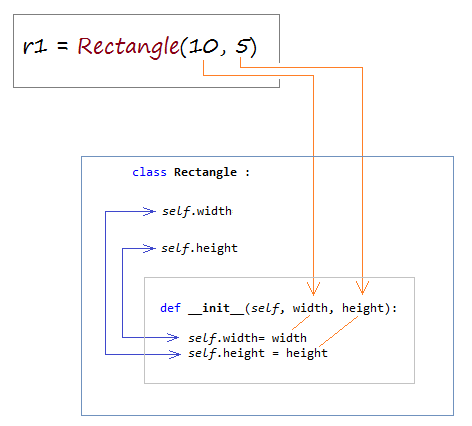3- Constructor with default parameters

Unlike other languages, the class in Python only has one constructor. However, Python allows the parameter to have a default value.
Note: All required parameters must precede all parameters with default values.
person.py

class Person :

# The age and gender parameters has a default value.
def __init__ (self, name, age = 1, gender = "Male" ):

self.name = name
self.age = age
self.gender= gender

def showInfo(self):

print ("Name: ", self.name)
print ("Age: ", self.age)
print ("Gender: ", self.gender)
For example:
testPerson.py

from person import Person

# Create an object of Person.
aimee = Person("Aimee", 21, "Female")

aimee.showInfo()

print (" --------------- ")

# default age, gender.
alice = Person( "Alice" )

alice.showInfo()

print (" --------------- ")

# Default gender.
tran = Person("Tran", 37)

tran.showInfo()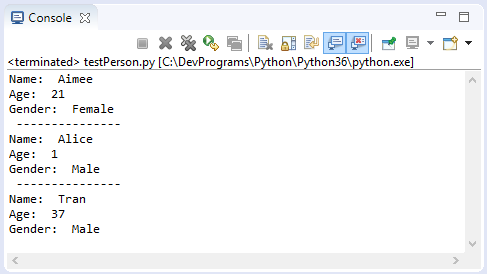4- Compare objects

In Python, when you create an object through Constructor, will be a real entity is created in memory, it has a specified address.

An operator assigned AA object by an BB  object does not create new entity on the memory, it's just pointing to address of AA to BB's address.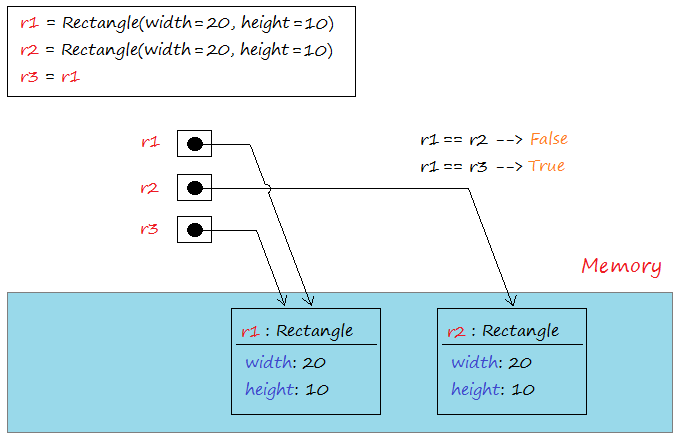== Operator used to compare two objects that is pointing to, it returns True if the two objects refer to the same address in memory. Operators != is used to compare the two addresses of two objects that is pointing to, it returns True if the two objects that point to two different addresses.
compareObject.py

from rectangle import Rectangle

r1 = Rectangle(20, 10)

r2 = Rectangle(20 , 10)

r3 = r1

# Compare the address of r1 and r2
test1 = r1 == r2 # --> False

# Compare the address of r1 and r2
test2 = r1 == r3 # --> True

print ("r1 == r2 ? ", test1)

print ("r1 == r3 ? ", test2)

print (" -------------- ")

print ("r1 != r2 ? ", r1 != r2)

print ("r1 != r3 ? ", r1 != r3)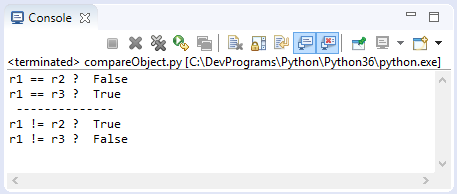5- Attributes

In Python, there are 2 similar concepts that you need to distinguish them:
1. Attribute
2. Class's variable
To simplify that, let's analyze the example below:
player.py

class Player:

# Class's Variable
minAge  = 18

maxAge = 50

def __init__(self, name, age):

self.name = name
self.age = age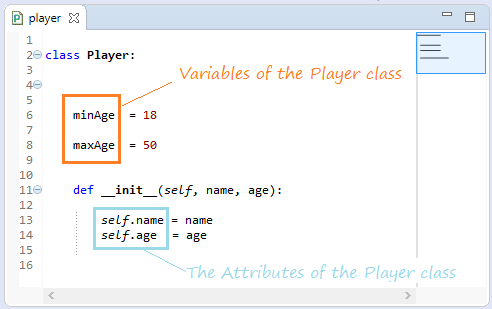Attribute
Objects created from one class will be located at different addresses on memory, and their "same name" properties also have different addresses on memory. For example: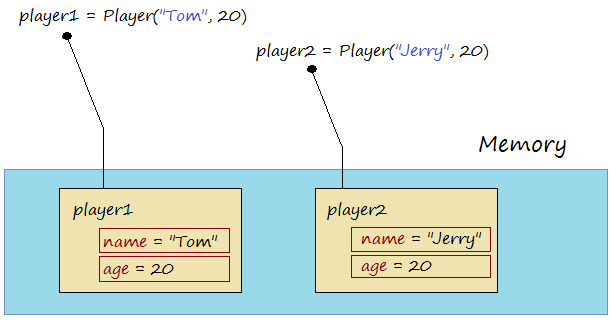testAttributePlayer.py

from player import Player

player1 = Player("Tom", 20)

player2 = Player("Jerry", 20)

print ("player1.name = ", player1.name)
print ("player1.age = ", player1.age)

print ("player2.name = ", player2.name)
print ("player2.age = ", player2.age)

print (" ------------ ")

print ("Assign new value to player1.age = 21 ")

# Assgin new value to age attribute of player1.
player1.age = 21

print ("player1.name = ", player1.name)
print ("player1.age = ", player1.age)

print ("player2.name = ", player2.name)
print ("player2.age = ", player2.age)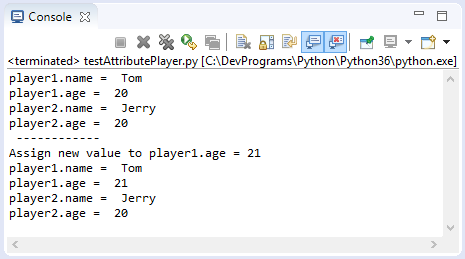Python allows you to create a new attribute for a pre-existing object. For example,  the player1 object and the new property named as address.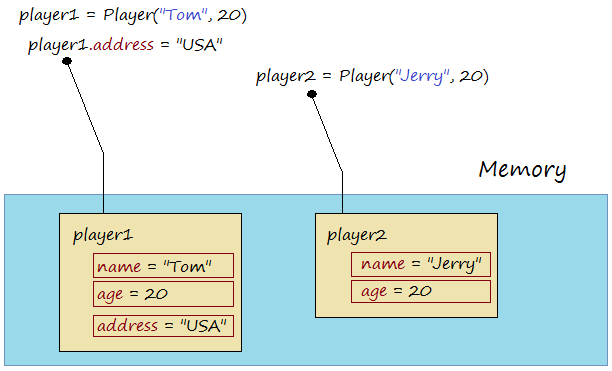testNewAttributePlayer.py

from player import Player

player1 = Player("Tom", 20)

player2 = Player("Jerry", 20)

# Create a new attribute named 'address' for player1.

print ("player1.name = ", player1.name)
print ("player1.age = ", player1.age)

print (" ------------------- ")

print ("player2.name = ", player2.name)
print ("player2.age = ", player2.age)

# player2 has no attribute 'address' (Error!!)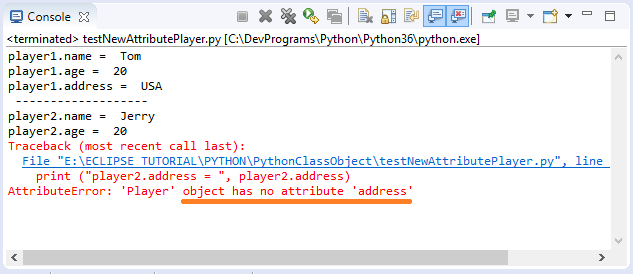6- Function Attributes

Normally, you access to the attribute of an object through the "dot" operator (e.g player1.name). However Python allows you to access to it via function.
 Function Discription getattr(obj, name[, default]) Returns the value of the attribute, or returns the default value if the object does not have this attribute. hasattr(obj,name) Check whether this object has an attribute given by the parameter 'name' or not. setattr(obj,name,value) Set value to attribute. If the attribute does not exist, it will be created. delattr(obj, name) Remove attribute.
testAttFunctions.py

from player import Player

player1 = Player("Tom", 20)

# getattr(obj, name[, default])
print ("getattr(player1,'name') = " , getattr(player1,"name") )

print ("setattr(player1,'age', 21): ")

# setattr(obj,name,value)
setattr(player1,"age", 21)

print ("player1.age = ", player1.age)

# Check player1 has 'address' attribute?

# Create attribute 'address' for object 'player1'
print ("Create attribute 'address' for object 'player1'")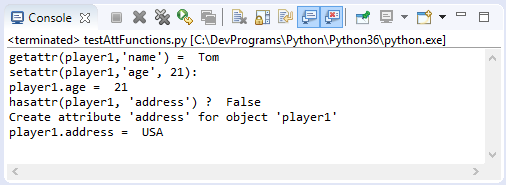7- Built-In Class Attributes

Python classes are descendants of the object class. So they inherit the following attributes:
 Attribute Description __dict__ Giving information about this class in a short, easy to understand, as one dictionary __doc__ Returns a string describing the class, or returns None if it is not defined __class__ Returns an object, containing information about the class, which has many useful attributes, including the __name__ attribute. __module__ Returns the 'module' name of the class, or returns "__main__" if that class is defined in the module being run.
testBuildInAttributes.py

class Customer :
'This is Customer class'

self.name = name
self.phone = phone

john = Customer("John",1234567, "USA")

print ("john.__dict__ = ", john.__dict__)

print ("john.__doc__ = ", john.__doc__)

print ("john.__class__ = ", john.__class__)

print ("john.__class__.__name__ = ", john.__class__.__name__)

print ("john.__module__ = ", john.__module__)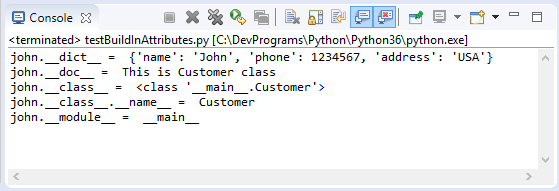8- Class's Variables

In Python, Class's Variable definition is equivalent to Static Field definition of other languages such as Java, CSharp. Variables of the class can be accessed via the class name or the object.The advice here is you should access to "Class's Variable" via the class name instead of the object. This helps to avoid confusion between "Class's Variable" and attribute.
Each of the Class's Variable has an address on memory. And it is shared with all the objects of class.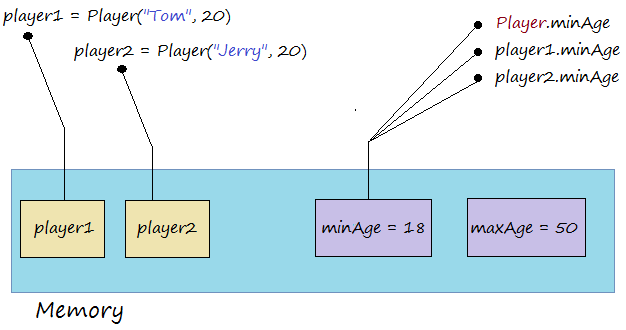testVariablePlayer.py

from player import Player

player1 = Player("Tom", 20)

player2 = Player("Jerry", 20)

# Access via class name.
print ("Player.minAge = ", Player.minAge)

# Access via object.
print ("player1.minAge = ", player1.minAge)
print ("player2.minAge = ", player2.minAge)

print (" ------------ ")

print ("Assign new value to minAge via class name, and print..")

# Assgin new value to minAge via class name
Player.minAge = 19

print ("Player.minAge = ", Player.minAge)
print ("player1.minAge = ", player1.minAge)
print ("player2.minAge = ", player2.minAge)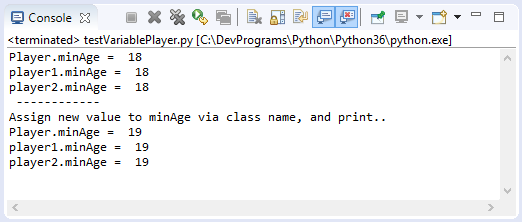9- List members of a class or object

Python provides you with the function dir, the function shows the list of the methods, attributes, variables of the class or object.
testDirFunction.py

from player import Player

# Print out list of attributes, methods and variables of class 'Player'
print ( dir(Player) )

print ("\n\n")

player1 = Player("Tom", 20)

# Print out list of attributes, methods and variables of object 'player1'
print ( dir(player1) )
Run the example:
['__class__', '__delattr__', '__dict__', '__dir__', '__doc__', '__eq__', '__format__', '__ge__', '__getattribute__', '__gt__', '__hash__', '__init__', '__init_subclass__', '__le__', '__lt__', '__module__', '__ne__', '__new__', '__reduce__', '__reduce_ex__', '__repr__', '__setattr__', '__sizeof__', '__str__', '__subclasshook__', '__weakref__', 'maxAge', 'minAge']

['__class__', '__delattr__', '__dict__', '__dir__', '__doc__', '__eq__', '__format__', '__ge__', '__getattribute__', '__gt__', '__hash__', '__init__', '__init_subclass__', '__le__', '__lt__', '__module__', '__ne__', '__new__', '__reduce__', '__reduce_ex__', '__repr__', '__setattr__', '__sizeof__', '__str__', '__subclasshook__', '__weakref__', 'address', 'age', 'maxAge', 'minAge', 'name']

10- Delete Object

• TODO

View more Tutorials:

Maybe you are interested

These are online courses outside the o7planning website that we introduced, which may include free or discounted courses.

•Python 3 For Beginner - Object-Oriented Programming
•Complete Python Web Course: Build 8 Python Web Apps
•Building User Interface Using React And Flux
•JDBC Servlets and JSP - Java Web Development Fundamentals
•Master Computer Vision™ OpenCV4 in Python with Deep Learning
•Learning Path: Python: Design and Architect Python Apps
•Learn JavaScript Fundamentals
•Learn iPython: The Full Python IDE
•Python 3000: Tactical SQL Quick-Start
•Python GUI : From A-to-Z With 2 Final Projects
•Learning iPython Notebook
•Python BeautifulSoup: Extract Web Data Beautifully
•Python Fundamentals
•Python MySql From Scratch
•Python Programming Full Course (Basics,OOP,Modules,PyQt)
•Learning Path: Python GUI Projects
•Starting with JavaScript Unit Testing
•Python 3000: Tactical File I/O
•Web Programming with Python
•Learning Path: Python Web Development
•New to Python Automation..?Try Step by Step Python 4 Testers
•Learn Programming in Python With the Power of Animation
•Python 2000: Beyond The Basics
•Java Spring and Hibernate:create a crud application